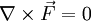Conservative force

Conservative forces are those that possess certain properties:

• The work it does on a particle is independent of its trajectory.
• The work done on a particle that moves along a closed trajectory (where the initial and final positions are the same, or di = df) = 0) is zero.
• The force can be written as the negative of the gradient of a potential energy function, i.e..
• The curl of the force,is zero,When the only forces present in a system are conservative, energy is conserved.

Examples of conservative forces include:

Friction is an example of a non-conservative force: